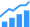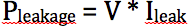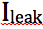# Low Power Design: RTL Power Analysis

Why it is so important to do the analysis at the RTL.In last month’s blog, we discussed and compared various power techniques. A quick recap of these power techniques is shown in figure 1. Selecting between them is often quite challenging. These techniques need to be selected during RTL design. At the RTL, designers need a power analysis solution that guides them to the right techniques for their design. In this month’s blog, we will review the power analysis background and show why it is important to do it at the RTL.

Power Analysis Background
It is well known that power consumed in a CMOS circuit can be calculated as the summation of dynamic power, leakage power and a small amount of instantaneous or short circuit power. Short circuit power is the power dissipation when both NMOS and PMOS transistors are ON at the same time. By controlling the slew rate, this power is minimized. Designer also know how to calculate the dynamic power consumed by a circuit as:where “C” is the capacitance, “V” is the operating voltage, “f” is the clock frequency, and “a” is the activity or percentage of the circuit toggling in a clock cycle.

Even though the equation seems easy, using this equation at the RTL is tricky. RTL designers need a lot of information regarding how the RTL will be implemented to estimate power correctly. We will see how this can be done in the next section.

Leakage power can be calculated as:where “V” is the operating voltage and “” is the leakage current. Leakage current is calculated for nodes that are not switching. Calculating which nodes are static can be tricky at the RTL.

Peak Power and Average Power
Designers need to analyze their designs for peak power and average power. Average power consumption should be below the power requirement set by the market. The power requirement is often specified as the Thermal Design Power (TDP) constraint. Package selection is also based on the average power consumption. So designers want to get this value early in their design cycle and make sure it is correlated with the final implementation.

Peak power typically happens when the design is subject to maximum activity. The peak power value is used to design the power supply. Peak power is also used to determine the power integrity. Higher peak power can cause excessive an IR drop, which will result in poor performance. Peak power can be handled by adding decoupling capacitances to the design. These capacitances store a charge during normal operation and supply the additional demand during peak operation. Designers also need to pay attention to the difference between peak power and average power. Too large a difference will require them to add a number of decoupling capacitances, which can increase the area and the leakage current. Too small a difference may point to missed optimization opportunities.

Today, SoCs operate across multiple modes with different IPs active at different modes. As a result, the difference between peak power and average power can be very large. Hence, power analysis at the RTL is important to identify and fix many of these issues.

Case for RTL Power Analysis
Computing peak power and average power helps us make important design decisions. It serves as a feasibility study if the design can work in the market of choice. It also lets us decide which power techniques are needed for the design. Once we decide on the power techniques, we can also estimate the overall schedule for the design implementation. In short, RTL power analysis is an important step for today’s designs. But, one of the key requirements is for the analysis to be as close to the gate level analysis as possible.
In the next month’s blog, we will discuss how we can get post-layout level accuracy in RTL power analysis.